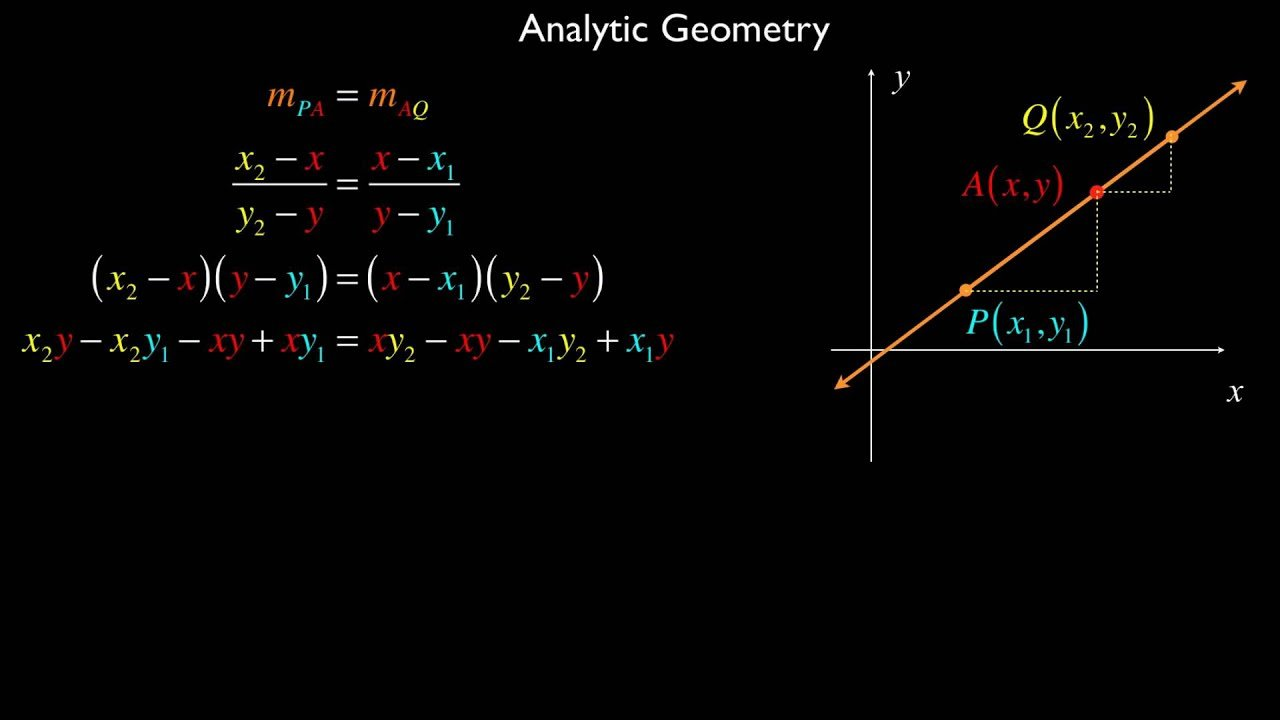Analytical Geometry Grade 12 Exercises: Questions and Answers pdf: In classical mathematics, analytic geometry, also known as coordinate geometry or Cartesian geometry, is the study of geometry using a coordinate system. This contrasts with synthetic geometry. Analytic geometry is used in physics and engineering, and also in aviation, rocketry, space science, and spaceflight.

Analytical Geometry Study Guide for Grade 12:

Analytical Geometry Study Guide with Questions and Answers (Mindset):

## Equations and curves

In analytic geometry, any equation involving the coordinates specifies a subset of the plane, namely the solution set for the equation, or locus. For example, the equation y = x corresponds to the set of all the points on the plane whose x-coordinate and y-coordinate are equal. These points form a line, and y = x is said to be the equation for this line. In general, linear equations involving x and y specify lines, quadratic equations specify conic sections, and more complicated equations describe more complicated figures.

Usually, a single equation corresponds to a curve on the plane. This is not always the case: the trivial equation x = x specifies the entire plane, and the equation x2 + y2 = 0 specifies only the single point (0, 0). In three dimensions, a single equation usually gives a surface, and a curve must be specified as the intersection of two surfaces (see below), or as a system of parametric equations. The equation x2 + y2 = r2 is the equation for any circle centered at the origin (0, 0) with a radius of r.View all #Mathematics-Grade 12 Study Resources

We have compiled great resources for Mathematics Grade 12 students in one place. Find all Question Papers, Notes, Previous Tests, Annual Teaching Plans, and CAPS Documents.

## More Grade 12 Mathematics Past Papers for Previous Years

Hey, Grade 12 Learners. My Courses portal has everything you needed to ace your matric exams, tests, assessments, research tasks, and assignments. Feel free to explore all resources for grade 12 learners, such as Study Guides, Department of Basic Education Past Exam Papers with Memos, and Speech Topics.